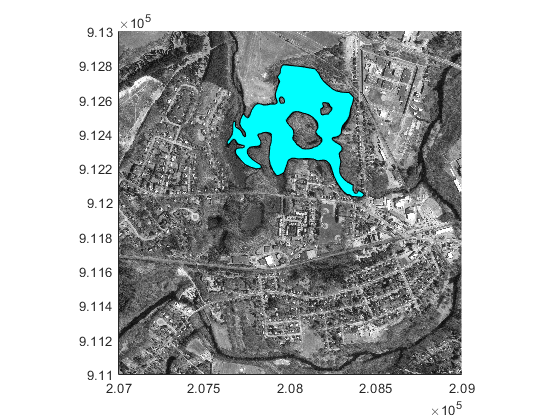# mappolyshape

Polygon in planar coordinates

## Description

A `mappolyshape` object represents a polygon or multipolygon in planar coordinates. A polygon is a region bounded by a closed curve and can include interior holes. A multipolygon is an individual polygon shape that includes multiple nonintersecting regions.

To represent a polygon or multipolygon in geographic coordinates, use a `geopolyshape` object instead.

## Creation

To create `mappolyshape` objects, either:

• Import polygon data in planar coordinates as a geospatial table using the `readgeotable` function, and then query the `Shape` variable of the table.

• Use the `mappolyshape` function (described here).

### Syntax

``shape = mappolyshape(x,y)``

### Description

example

````shape = mappolyshape(x,y)` creates a `mappolyshape` object or array of `mappolyshape` objects with vertices at the specified x- and y-coordinates. The sizes of `x`, `y`, and the `mappolyshape` object array `shape` match.The `mappolyshape` function assumes that `x` and `y` define polygons with valid topology. A polygon has valid topology when: Region interiors are to the right as you trace boundaries from vertex to vertex.The boundaries have no self-intersections. In general, the outer boundaries of polygons with valid topology have vertices in a clockwise order and the interior holes have vertices in a counterclockwise order.```

### Input Arguments

expand all

x-coordinates, specified as a numeric vector or a cell array of numeric vectors.

• Create a polygon by specifying a vector, such as ```[-113 -49 -100 -113]```.

• Create a polygon with holes or a multipolygon by specifying a vector and including breaks between the hole and region boundaries as `NaN` values, such as ```[69 90 105 79 69 NaN 6 52 43 14 6 NaN 18 32 22 18]```.

• Create an array of polygons and multipolygons by specifying a cell array of vectors, such as ```{[69 90 105 79 69],[6 52 43 14 6 NaN 18 32 22 18]}```.

The `NaN` values in `x` must correspond to the `NaN` values in `y`.

The size of `x` must match the size of `y`. For cell arrays, the size of the vector in each cell of `x` must match the size of the vector in the corresponding cell of `y`.

Data Types: `double` | `cell`

y-coordinates, specified as a numeric vector or a cell array of numeric vectors.

• Create a polygon by specifying a vector, such as ```[39 45 19 39]```.

• Create a polygon with holes or a multipolygon by specifying a vector and including breaks between the hole and region boundaries as `NaN` values, such as ```[37 46 31 20 37 NaN 45 49 35 32 45 NaN 35 40 42 35]```.

• Create an array of polygons and multipolygons by specifying a cell array of vectors, such as ```{[37 46 31 20 37],[45 49 35 32 45 NaN 35 40 42 35]}```.

The `NaN` values in `x` must correspond to the `NaN` values in `y`.

The size of `x` must match the size of `y`. For cell arrays, the size of the vector in each cell of `x` must match the size of the vector in the corresponding cell of `y`.

## Properties

expand all

Number of regions, returned as an array of nonnegative integers. A region is a connected area such that any two points within the area can be connected by a path entirely within the area. Regions may contain holes.

For a `mappolyshape` scalar, the value of `NumRegions` is `1` when the `mappolyshape` object represents a single polygon and more than `1` when the object represents a multipolygon.

For a `mappolyshape` array, the size of `NumRegions` matches the size of the array.

Data Types: `double`

Number of holes in the polygon or multipolygon, returned as an array of nonnegative integers.

For a `mappolyshape` array, the size of `NumHoles` matches the size of the array.

Data Types: `double`

Geometric type, returned as `"polygon"`.

Data Types: `string`

Coordinate system type, returned as `"planar"`.

Data Types: `string`

Projected coordinate reference system (CRS), specified as a `projcrs` object. A projected CRS consists of a geographic CRS and several parameters that are used to transform coordinates to and from the geographic CRS.

## Examples

collapse all

Import a shapefile containing hydrographic data for Concord, MA as a geospatial table. The shapefile represents the data using polygons.

`hydro = readgeotable("concord_hydro_area.shp");`

Create a subtable that contains a polygon representing a pond. Get information about the polygon by querying the `Shape` variable of the table.

```pond = hydro(14,:); pond.Shape```
```ans = mappolyshape with properties: NumRegions: 1 NumHoles: 3 Geometry: "polygon" CoordinateSystemType: "planar" ProjectedCRS: [1×1 projcrs] ```

Import an orthophoto of Concord, MA, along with a map cells reference object and a colormap. Display the orthophoto and polygon on a map.

```[ortho,R,cmap] = readgeoraster("concord_ortho_w.tif"); mapshow(ortho,cmap,R) mapshow(pond,FaceColor="c")```Create an individual polygon with no holes as a `mappolyshape` scalar. Specify the projected CRS as World Equidistant Cylindrical, which has the EPSG authority code `4087`.

```x = [-113 -49 -100 -113]; y = [39 45 19 39]; polyshp = mappolyshape(x,y); p = projcrs(4087); polyshp.ProjectedCRS = p```
```polyshp = mappolyshape with properties: NumRegions: 1 NumHoles: 0 Geometry: "polygon" CoordinateSystemType: "planar" ProjectedCRS: [1x1 projcrs] ```

Create a multipolygon with two regions and one hole as a `mappolyshape` scalar.

```x = [69 90 105 79 69 NaN 6 52 43 14 6 NaN 18 32 22 18]; y = [37 46 31 20 37 NaN 45 49 35 32 45 NaN 35 40 42 35]; multipolygon = mappolyshape(x,y); multipolygon.ProjectedCRS = p```
```multipolygon = mappolyshape with properties: NumRegions: 2 NumHoles: 1 Geometry: "polygon" CoordinateSystemType: "planar" ProjectedCRS: [1x1 projcrs] ```

Create two individual polygons as a 1-by-2 `mappolyshape` array. The second polygon contains a hole.

```x = {[69 90 105 79 69],[6 52 43 14 6 NaN 18 32 22 18]}; y = {[37 46 31 20 37],[45 49 35 32 45 NaN 35 40 42 35]}; polyArray = mappolyshape(x,y); polyArray.ProjectedCRS = p```
```polyArray=1×2 object 1x2 mappolyshape array with properties: NumRegions: [1 1] NumHoles: [0 1] Geometry: "polygon" CoordinateSystemType: "planar" ProjectedCRS: [1x1 projcrs] ```

### Topics

Introduced in R2021b

## SupportGet trial now